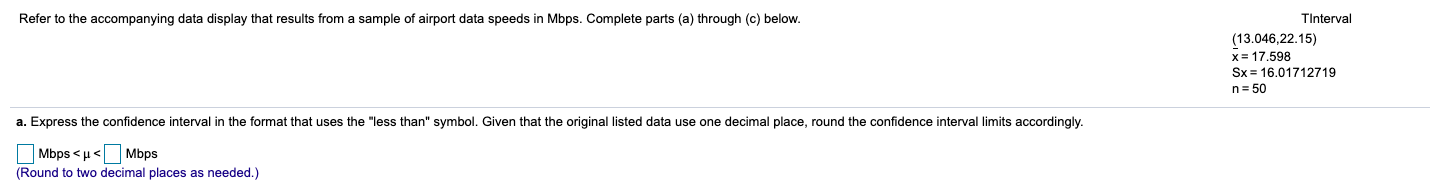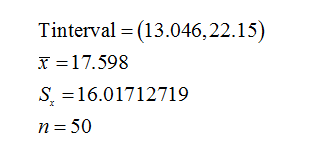# Refer to the accompanying data display that results from a sample of airport data speeds in Mbps. Complete parts (a) through (c) below.TInterval(13.046,22.15)x= 17.598Sx = 16.01712719n= 50a. Express the confidence interval in the format that uses the "less than" symbol. Given that the original listed data use one decimal place, round the confidence interval limits accordingly.O Mbps < u<(Round to two decimal places as needed.)Mbps

Question
66 viewshelp_outlineImage TranscriptioncloseRefer to the accompanying data display that results from a sample of airport data speeds in Mbps. Complete parts (a) through (c) below. TInterval (13.046,22.15) x= 17.598 Sx = 16.01712719 n= 50 a. Express the confidence interval in the format that uses the "less than" symbol. Given that the original listed data use one decimal place, round the confidence interval limits accordingly. O Mbps < u< (Round to two decimal places as needed.) Mbps fullscreen
check_circle

Step 1

Given:...

### Want to see the full answer?

See Solution

#### Want to see this answer and more?

Solutions are written by subject experts who are available 24/7. Questions are typically answered within 1 hour.*

See Solution
*Response times may vary by subject and question.
Tagged in